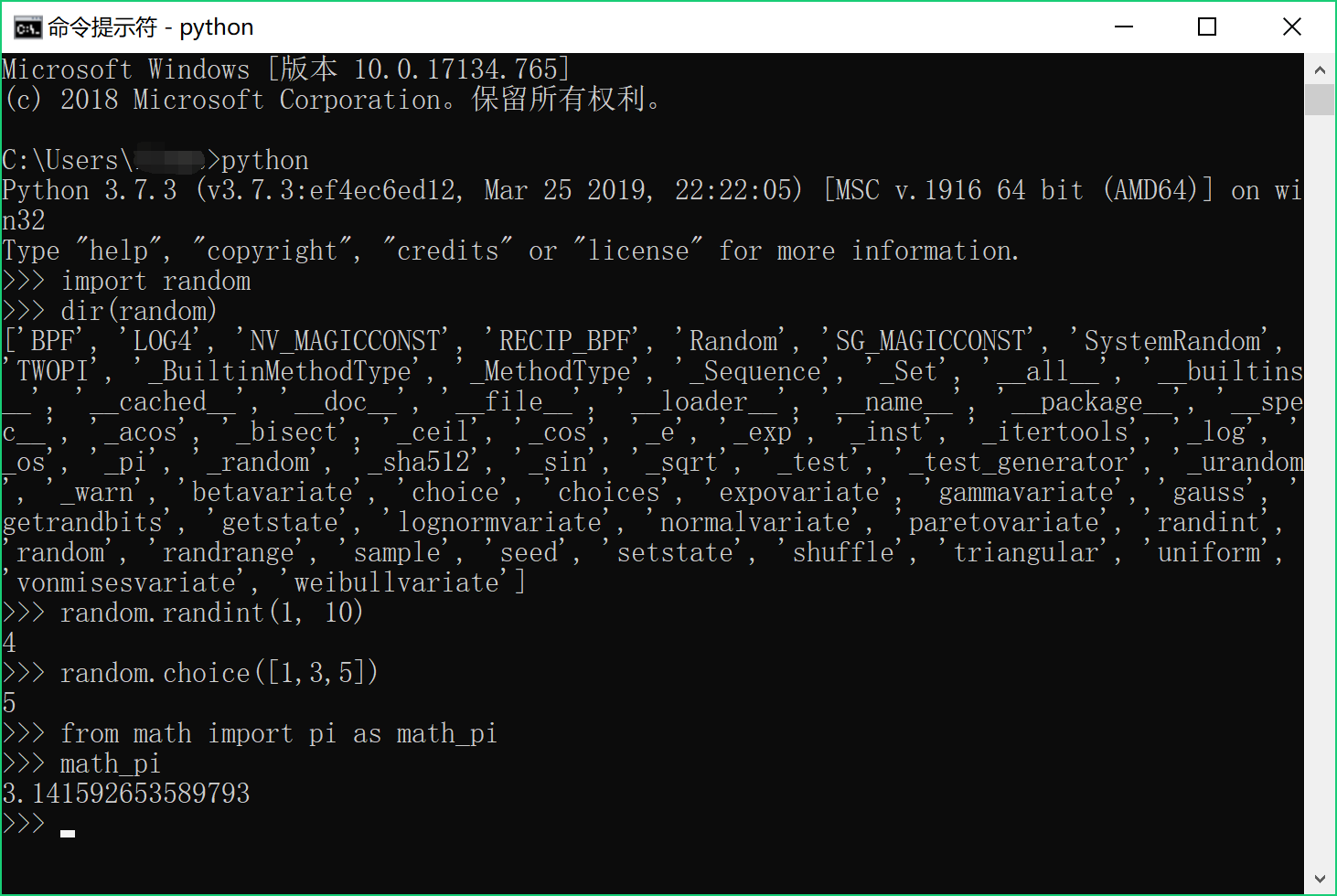## 【Python 第38课】模块

-- 牛顿

python自带了功能丰富的标准库，另外还有数量庞大的各种第三方库。使用这些“巨人的”代码，可以让开发事半功倍，就像用积木一样拼出你要的程序。

``import random``

import语句告诉python，我们要用random模块中的内容。然后便可以使用random中的方法，比如：

``````random.randint(1, 10)
random.choice([1, 3, 5])``````

``dir(random)``

``````from math import pi
print (pi)``````

``````from math import pi as math_pi
print (math_pi)``````